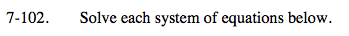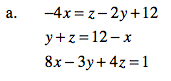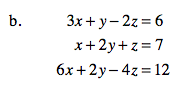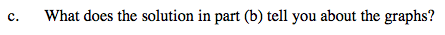Home > A2C > Chapter 7 > Lesson 7.2.1 > Problem7-102

7-102.
1. Solve each system of equations below. Homework Help ✎

1. −4x = z − 2y + 12
y + z = 12 − x
8x − 3y + 4z = 1

2. 3x + y − 2z = 6
x + 2y + z = 7
6x + 2y − 4z = 12

3. What does the solution in part (b) tell you about the graphs?Reorganize the equations so all of the variable terms are on one side and the constants are on the other side.

For additional help refer to problem 7-50.See part (a).

There are infinite solutions.Think about what this solution would mean in two dimensions. Would this mean the same thing in three dimensions?